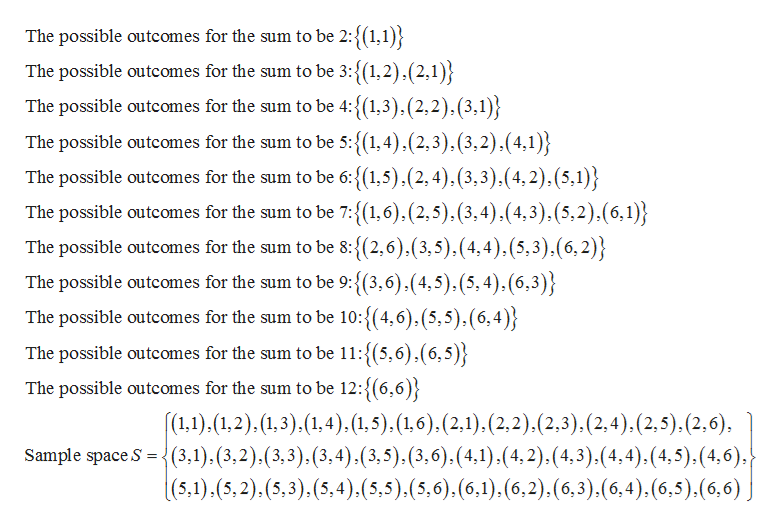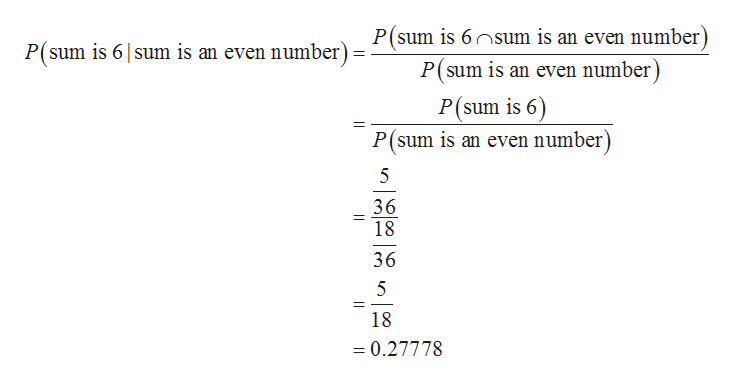# Two fair dice are rolled at once. Find the conditional probability that the sum is 6, given that the sum is an even number.

Question

Two fair dice are rolled at once. Find the conditional probability that the sum is 6, given that the sum is an even number.

check_circleExpert Solution
Step 1

Concept of conditional probability:

For any two events A and B with P(B) >0, the formula for conditional probability is given by:

Step 2

Find the sample space:

Sample space:

The set of all possible outcomes of a probability experiment is called sample space of the experiment.

Here, two fair dice are rolled.

The total number of outcomes in the sample space be n(S) = 62 = 36. That is, there will be 36 equally likely outcomes.

Outcomes will be occurred in ordered pairs. First number in each ordered pair represents the number on the first die and second number in each ordered pair represents the number on the second die. Each of the two numbers can take values 1 to 6.

The sum of the numbers on the dice will be 2, 3, 4, 5, 6, 7, 8, 9, 10,11 and 12.

The sample space for the experiment is given below:help_outlineImage TranscriptioncloseThe possible outcomes for the sum to be 2:{(1,1)} The possible outcomes for the sum to be 3:{(1,2),(2,1) The possible outcomes for the sum to be 4:{(1,3).(2,2),(3,1 The possible outcomes for the sum to be 5:{1,4),(2,3).(3,2).(4,1 The possible outcomes for the sum to be 6: {(1,5),(2,4),(3,3).(4,2), (5,1)} The possible outcomes for the sum to be 7:{(1,6).(2,5).(3,4).(4,3). (5,2).(6,1) The possible outcomes for the sum to be 8:{(2,6).(3,5), (4,4),(5,3).(6,2)} The possible outcomes for the sum to be 9:{(3,6),(4,5).(5,4),(6,3) The possible outcomes for the sum to be 10:{(4,6),(5,5),(6,4) The possible outcomes for the sum to be 11:{(5,6).(6,5)} The possible outcomes for the sum to be 12:{(6,6) (1,1).(1,2), (1,3).(1.4).(1.5).(1,6).(2,1).(2,2).(2,3).(2,4).(2,5).(2,6), Sample space S (3,1), (3,2).(3,3), (3,4),(3,5).(3,6).(4,1).(4,2).(4,3).(4,4),(4,5).(4,6), |(5,1).(5,2),(5,3), (5,4),(5,5).(5,6).(6,1).(6,2),(6,3).(6,4),(6,5).(6,6) fullscreen
Step 3

Find the conditional probability that the sum is 6, given that the sum is an even number:

There are 5 different ways the sum of the two dice is 6 and 18 different ways the sum of the two dice is an ...help_outlineImage TranscriptioncloseP(sum is 6sum is an even number) P(sum is an even number P(sum is 6 sum is an even number)= P(sum is 6) P(sum is an even number) 5 36 18 36 5 18 0.27778 fullscreen

### Want to see the full answer?

See Solution

#### Want to see this answer and more?

Solutions are written by subject experts who are available 24/7. Questions are typically answered within 1 hour*

See Solution
*Response times may vary by subject and question
Tagged in

### Probability